Next: 4.2 Classical-to-fractal geometric transition Up: 4. Fractal Strings Previous: 4. Fractal Strings

## 4.1 The Hausdorf dimension of a quantum string

One of the major achievements of Feynman's formulation of quantum mechanics was to restore the particle's trajectory concept at the quantum level. However, the dominant contribution to the sum over histories'' is provided by trajectories which are nowhere differentiable . Non differentiability is the hallmark of fractal lines. In fact, it seems that Feynman and Hibbs were aware that the quantum mechanical path of a particle is inherently fractal. This idea was revisited, and further explored by Abbott and Wise in the case of a free non-relativistic particle , and the extension to relativistic particles was carried out by several authors, but without a general agreement . This is one of the reasons for setting up a quantum mechanical, rather than a field theoretical, framework, even for relativistic objects. It enables us to adapt the Abbott-Wise discussion to the string case, with the following basic substitution: the point particle, erratically moving through euclidean space, is replaced by the string configuration whose representative point randomly drifts through loop space. We have shown in the previous sections that flow of time'' for particles is replaced by area variations for strings. Hence, the image of an abstract linear trajectory'' connecting the two points'' C 0 and C in a lapse of time A, corresponds, physically, to a family of closed lines stacked into a two-dimensional surface of proper area A. Here is where the quantum mechanical aspect of our approach, and our choice of dynamical variables, seem to have a distinct advantage over the more conventional relativistic description of string dynamics. The conventional picture of a string world-surface, consisting of a collection of world-lines associated with each constituent point, is replaced by a world-sheet foliation'' consisting of a stack of closed lines labelled by the internal parameter A. In other words, we interpret the string world-surface as a sequence of snapshots'' of single closed lines ordered with respect to the interior area bounded by them. Then, the randomness of the motion'' of a point in loop space is a reflection of the non-differentiability of the string world-sheet, which, in turn, is due to zero-point quantum fluctuations: the random addition of petals to each loop results in a fuzziness, or graininess of the world surface, by which the string stack acquires an effective thickness. One can expect that this graininess becomes apparent only when one can resolve the surface small irregularities.
The technical discussion on which this picture is based, follows closely the analysis by Abbott and Wise . Thus, let us divide the string internal coordinates domain into N strips of area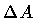. Accordingly, the string stack is approximated by the discrete set of the N + 1 loops,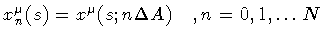. Suppose now that we take a snapshot of each one of them, and measure their internal area. If the cross section of the emulsion grains is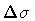, then we have an area indeterminacy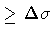. Then, the total area of the surface subtended by the last and first loop in the stack will be given by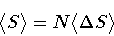(38)

where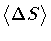is the average area variation in the interval,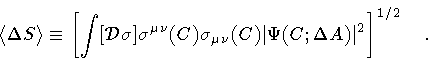(39)

The finite resolution in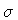is properly taken into account by choosing a gaussian wave functional of the type (23) for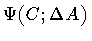. Next, following our correspondence code between particles and strings, we define the Hausdorff measure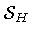for the stack of fluctuating loops through the relation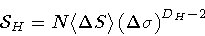(40)

where D H is a number determined by the requirement that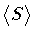be independent of the resolution.
In the above calculation, we may represent the quantum state of the string by the loop functional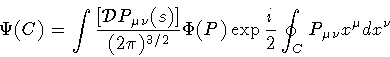(41)

with a gaussian momentum distributioncentered around a vanishing string average momentum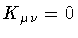, i.e., for the moment, we consider a free loop subject only to zero-point fluctuations. Then,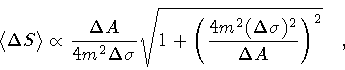(42)

and, in order to determine the string fractal dimension we keepfixed and take the limit: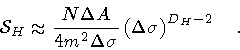(43)

Hence, in order to eliminate the dependence on,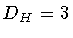. As in the point particle case, quantum fluctuations increase by one unit the dimension of the string classical path. As discussed in the following subsection, the gradual appearance of an extra dimension is perceived as fuzziness of the string manifold, and the next question to be addressed is which parameter, in our quantum mechanical approach, controls the transition from classical to fractal geometry.Next: 4.2 Classical-to-fractal geometric transition Up: 4. Fractal Strings Previous: 4. Fractal Strings